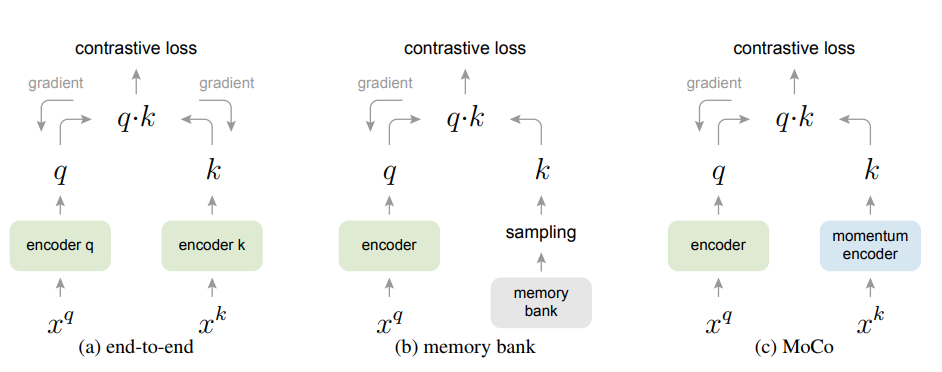## 对比学习

${L}_{q}=-\mathrm{log}\frac{\mathrm{exp}\left(q\cdot {k}_{+}/\tau \right)}{\sum _{i=0}^{K}\mathrm{exp}\left(q\cdot {k}_{i}/\tau \right)}\phantom{\rule{0ex}{0ex}}$

## Moco

${\theta }_{k}←m{\theta }_{k}+\left(1-m\right){\theta }_{q}\phantom{\rule{0ex}{0ex}}$

Moco的核心就是维护一个动态的queue作为字典，然后通过momentum update方式更新字典keys编码网络，其PyTorch伪代码如下所示：

# f_q, f_k: encoder networks for query and key
# queue: dictionary as a queue of K keys (CxK)
# m: momentum
# t: temperature

f_k.params = f_q.params # initialize
for x in loader: # load a minibatch x with N samples
x_q = aug(x) # a randomly augmented version
x_k = aug(x) # another randomly augmented version

q = f_q.forward(x_q) # queries: NxC
k = f_k.forward(x_k) # keys: NxC
k = k.detach() # no gradient to keys

# positive logits: Nx1
l_pos = bmm(q.view(N,1,C), k.view(N,C,1))

# negative logits: NxK
l_neg = mm(q.view(N,C), queue.view(C,K))

# logits: Nx(1+K)
logits = cat([l_pos, l_neg], dim=1)

# contrastive loss, Eqn.(1)
labels = zeros(N) # positives are the 0-th, so GT label is 0
loss = CrossEntropyLoss(logits/t, labels)

# SGD update: query network
loss.backward()
update(f_q.params)

# momentum update: key network
f_k.params = m*f_k.params+(1-m)*f_q.params

# update dictionary
enqueue(queue, k) # enqueue the current minibatch
dequeue(queue) # dequeue the earliest minibatch
# bmm: batch matrix multiplication; mm: matrix multiplication; cat: concatenation


augmentation = [
transforms.RandomResizedCrop(224, scale=(0.2, 1.)),
transforms.RandomGrayscale(p=0.2),
transforms.ColorJitter(0.4, 0.4, 0.4, 0.4),
transforms.RandomHorizontalFlip(),
transforms.ToTensor(),
normalize
]


# compute query features，query计算过程不做改动
q = self.encoder_q(im_q)  # queries: NxC
q = nn.functional.normalize(q, dim=1)

# key的计算要先shuffle 样本，这样同一张上query和key的BN层采用不同的样本计算得到，避免信息泄露
# shuffle for making use of BN
im_k, idx_unshuffle = self._batch_shuffle_ddp(im_k)

k = self.encoder_k(im_k)  # keys: NxC
k = nn.functional.normalize(k, dim=1)

# undo shuffle
k = self._batch_unshuffle_ddp(k, idx_unshuffle)


augmentation = [
transforms.RandomResizedCrop(224, scale=(0.2, 1.)),
transforms.RandomApply([
transforms.ColorJitter(0.4, 0.4, 0.4, 0.1)  # not strengthened
], p=0.8),
transforms.RandomGrayscale(p=0.2),